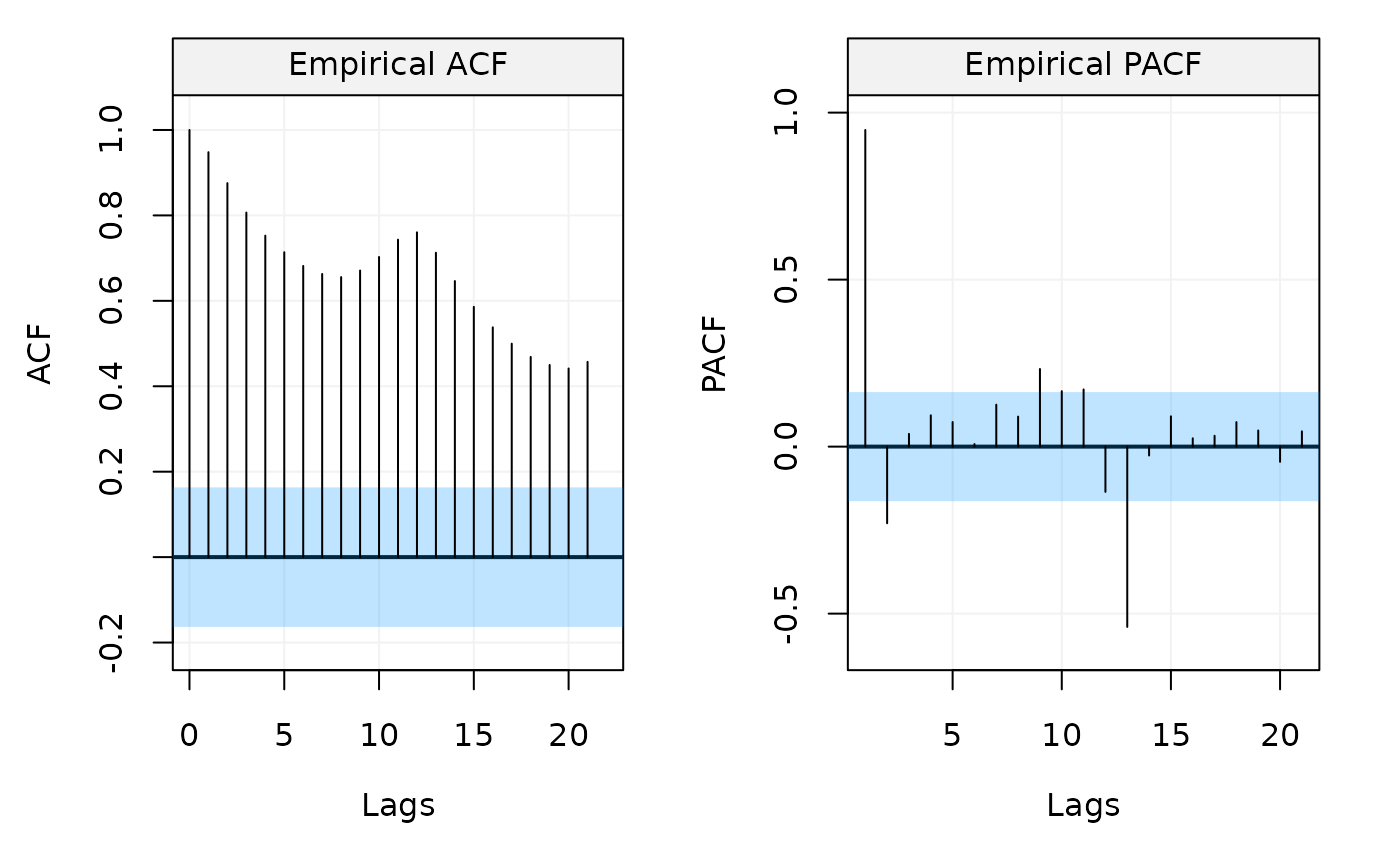Correlation Analysis function computes and plots both empirical ACF and PACF of univariate time series.

corr_analysis(
x,
lag.max = NULL,
type = "correlation",
demean = TRUE,
show.ci = TRUE,
alpha = 0.05,
plot = TRUE,
...
)

## Arguments

x

A vector or "ts" object (of length $$N > 1$$).

lag.max

A integer indicating the maximum lag up to which to compute the ACF and PACF functions.

type

A character string giving the type of acf to be computed. Allowed values are "correlation" (the default) and "covariance".

demean

A bool indicating whether the data should be detrended (TRUE) or not (FALSE). Defaults to TRUE.

show.ci

A bool indicating whether to compute and show the confidence region. Defaults to TRUE.

alpha

A double indicating the level of significance for the confidence interval. By default alpha = 0.05 which gives a 1 - alpha = 0.95 confidence interval.

plot

A bool indicating whether a plot of the computed quantities should be produced. Defaults to TRUE.

...

## Value

Two array objects (ACF and PACF) of dimension $$N \times S \times S$$.

Yunxiang Zhang

## Examples

# Estimate both the ACF and PACF functions
corr_analysis(datasets::AirPassengers)